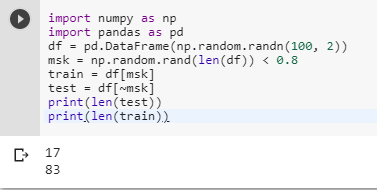2 views
in Python

I have a fairly large dataset in the form of a dataframe and I was wondering how I would be able to split the data frame into two random samples (80% and 20%) for training and testing.

Thanks!

by (106k points)

To create test and train samples from one dataframe with pandas it is recommended to use  numpy's randn:

import numpy as np

import pandas as pd

df = pd.DataFrame(np.random.randn(100, 2))

msk = np.random.rand(len(df)) < 0.8

train = df[msk]

test = df[~msk]

print(len(test))

print(len(train))+1 vote